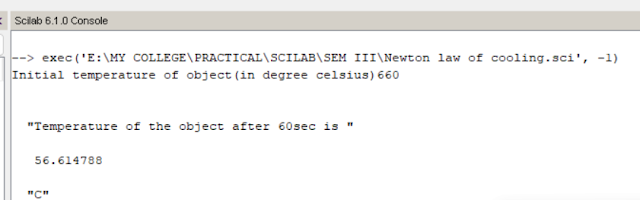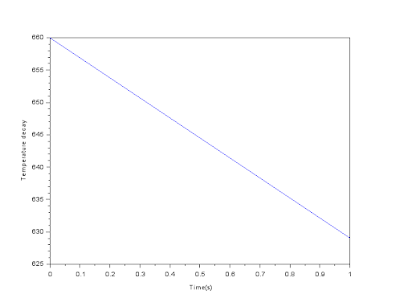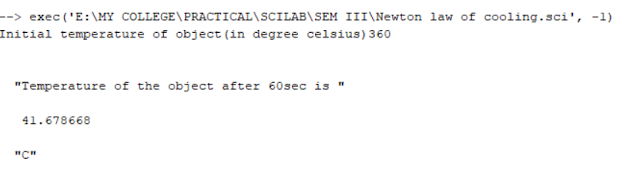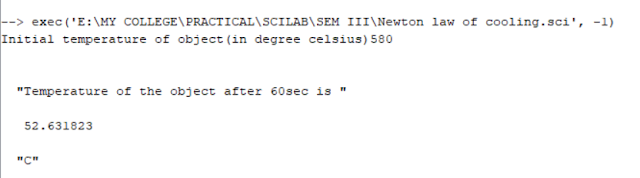Verify Newton's law of cooling for a rectangular iron bar of dimension 25cmX7cmX4cm having mass 7kg & with initial temperature of 660C. Repeat the experiment with three more sets of parameters of your choice so as to prove the law.

AIM OF THE EXPERIMENT:

To verify Newton's law of cooling for a rectangular iron bar of dimension 25cmX7cmX4cm having mass 7kg & with initial temperature of 660C. Repeat the experiment with three more sets of parameters of your choice so as to prove the law.

A PC/laptop.

Scilab 6.1.0 must be installed.
THEORY:
Newton's law of cooling states that the rate of heat loss of a body is directly proportional to the difference of temperature between the body & its surrounding.

T(t) = Ts + (To – Ts) e-kt

Where,

t = time,

T(t) = Temperature of the given body at time t,

Ts = Surrounding temperature,

To = Initial temperature of the body,

k = Cooling constant.

CODING:

```// Defining the variables
T0=input("Initial temperature of object(in degree celsius)")
Ts=25;          //Temperature of the surroundings in degree celsius
k=0.05;         //constant
t=0:1:1;        //Time Interval in sec

//Computation of Newton's Law of cooling
Tt=Ts+(T0-Ts)*exp(-k*t);

//Plotting the graph
plot(t,Tt);
xlabel("Time(s)");
ylabel("Temperature decay");

//Temperature after 60sec
Tt=Ts+(T0-Ts)*exp(-k*60);
disp("Temperature of the object after 60sec is ",Tt,"C");```
`OUTPUT:`
``